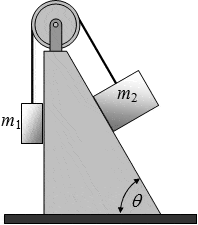# Solve 2-Mass Pulley System Homework for Moving Blocks

• dgZeyas
In summary, the tension in the string is Wsinθ/f, where f is the static friction. If block 1 is heavier than block 2, then block 2 will move up the ramp.

## Homework Statement

A massless string, passing over the frictionless pulley, connects the two masses as shown. Block m1 hangs vertically without touching the wall, but m2 slides along the wall with friction (μs = 0.61, and μk = 0.14). If θ = 53°, how many times heavier than block 1 must block 2 be to start the system moving?## Homework Equations

1. ƩFx = max and ƩFy = may

2. fs = μsN
3. fk = μkN

## The Attempt at a Solution

I set up a free body diagram for m2, oriented as it is in space, such that the positive x-direction points up the slope and the positive y-direction points perpendicularly out of the slope. Then the forces are as follows:

T (tension): + x-direction
W (weight): m2gsinθ in the -x-direction and m2gcosθ in the -y-direction
N (normal): +y direction
fs (static friction): +x-direction (when m2 is NOT moving)
fk (static friction): -x-direction (when m2 IS moving)

So I figured the static friction would be this:

fs= μs(m2)(g)(sinθ)

as per equation 2.

But this is about as far as I got. I'm trying to find how many times heavier than block 1 must block 2 be to start the system moving-- I inferred from this that block 2 would move UP the ramp if block 1 was heavier, which makes sense, and while I have an equation containing m2, I'm having trouble figuring out another equation so a ratio can be set up.

Any help appreciated, thanks in advance!

Last edited:
dgZeyas said:
So I figured the static friction would be this:

fs= μs(m2)(g)(sinθ)

as per equation 2.
(Emphasis in red, mine.)

Are you sure about that? (Hint: what's the normal force on m2?)

After that, the next step is to ask yourself, "if nothing is moving, what is the tension in the string?"

Oh, yeah, ok it'd be cosθ. Because the normal force is the positive y-component of the weight. Thanks for pointing that out.

Tension (according to my coordinate system) is in the positive x-direction. OK. So if nothing is moving, ƩFx=0, as per equation 2 and Newton's second law.

So ƩFx = T + f - Wsinθ = 0, where W is the weight of the second mass.

So T = Wsinθ/f. f is the static friction ,or μs multiplied by the normal force... which is a vector in the y-direction, not the x, so now I confused how to add that bit in.

Thanks for the pointers so far.

Edit: I got a bit further and I can calculate the tension in the string using a FBD for mass 1. So that would enable me to find the static friction force, but I don't particularly see how that gets me closer to the answer either to be honest.

Last edited:
dgZeyas said:
Oh, yeah, ok it'd be cosθ. Because the normal force is the positive y-component of the weight. Thanks for pointing that out.

Tension (according to my coordinate system) is in the positive x-direction. OK. So if nothing is moving, ƩFx=0, as per equation 2 and Newton's second law.

So ƩFx = T + f - Wsinθ = 0, where W is the weight of the second mass.

So T = Wsinθ/f. f is the static friction ,or μs multiplied by the normal force... which is a vector in the y-direction, not the x, so now I confused how to add that bit in.

Thanks for the pointers so far.

Edit: I got a bit further and I can calculate the tension in the string using a FBD for mass 1. So that would enable me to find the static friction force, but I don't particularly see how that gets me closer to the answer either to be honest.

You're doing great!But don't forget! You've already found an expression for the static friction force in your first post (with the cos correction in your last post),
fs = μsm2g cosθ.
So feel free to substitute that in.

You've also mentioned that by using mass m1 that you can also find another expression with the tension in it, so that get's rid of the T variable.

Now just express m2/m1 as a function of θ and μs, and that's pretty much it.

Last edited:
Oh, and welcome to Physics Forums, by the way!

Long story short-- I've gotten it all figured out. As my professor says, non-calc physics is just a LOT of algebra. Well, yes it is, yes it is.